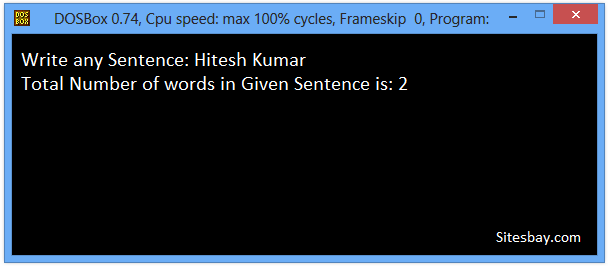# C++ Program to Count Word in Given Sentence

## C++ Program to Find Number of Words in Given Sentence

To Find Number of Words in Given Sentence in C++ program we need to enter any Sentence for user side. After enter sentence count total number of words present in the given string, next search for spaces, if found any space then increase a variable say countword by 1 and continue to check for next space.

To understand below example, you have must knowledge of following C++ programming topics; For Loop in C++, Cin Function in C++ and Cout Function in C++.

## Count Word in Given Sentence Program in C++

```#include<iostream.h>
#include<conio.h>
#include<string.h>
#include<stdio.h>
void main()
{
clrscr();
char strs, countword=0, strword;
int i, len;
cout<<"Write any Sentence: ";
gets(strs);
len=strlen(strs);
for(i=0; i<len; i++)
{
if(strs[i]==' ')
{
countword++;
}
}
cout<<"Total Number of words in Given Sentence is: "<<countword+1;
getch();
}
```

## Output

```Write any Sentence: Hitesh Kumar
Total Number of words in Given Sentence is: 2
```